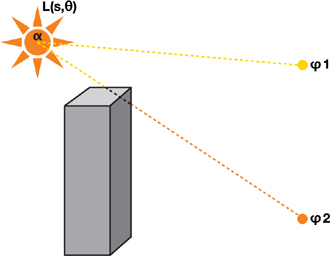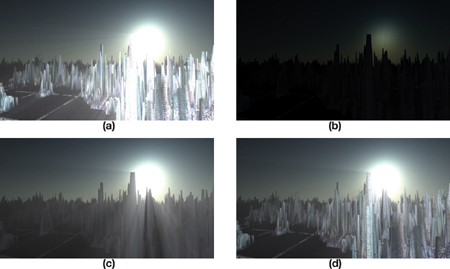# 体积散射

$L(s, \theta)=L_{0} e^{-\beta_{ex} s}+\frac{1}{\beta_{\mathrm{ex}}} E_{\text {sun }} \beta_{\mathrm{sc}}(\theta)\left(1-e^{-\beta_{ex} s}\right)$$L(s,\theta,\phi) = (1 - D(\phi))L(s,\theta)$

$L(s, \theta, \phi)=\sum_{i=0}^{n} \frac{L\left(s_{i}, \theta_{i}\right)}{n}$

$L(s, \theta, \phi)=\text { exposure } \times \sum_{i=0}^{n} \text { decay }{ }^{i} \times \text { weight } \times \frac{L\left(s_{i}, \theta_{i}\right)}{n}$

# 屏幕空间遮蔽方法

## 遮蔽 Pre-Pass 方法